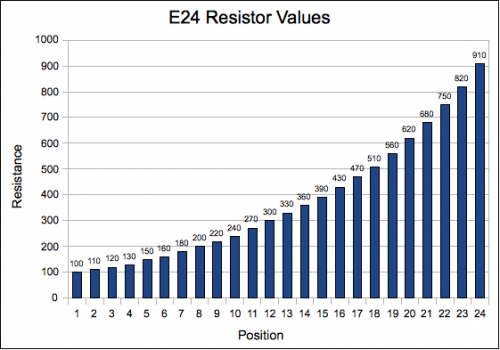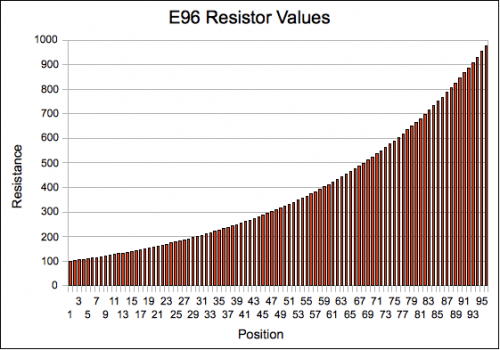# EIA Resistor Values Explained

Have you ever wondered why standard 5% resistors have strange values, like 330 and 470 Ohms, instead of nice round numbers like 300 or 500 Ohms?

It turns out that standard resistor values form a preferred number series defined by the EIA.  5% values are part of a standard called E24.  The standard is based on a geometric series – each value is approximately 1.1 times the previous one in the set.

This scheme ensures that the resistance values are separated by an amount approximately equal to twice their tolerance.  Since a 5% tolerance resistor could actually be plus or minus 5% of the nominal value, the E24 range spaces the values by 10%.  That way, where the tolerance range of one value leaves off, the next higher value picks up, with the smallest possible overlap or gaps in resistance.

For example, 330 Ohms + 5% = 347 Ohms.  The next highest E24 value is 360 ohms, and 360 Ohms – 5% = 342 Ohms.  There is a small overlap of 5 ohms because the values don’t follow the geometric series exactly (due to rounding to the nearest 10 Ohms).  Spacing resistances significantly closer than their tolerance range would be silly – a 330 Ohm resistor could in reality be larger than a resistor marked 335 Ohms if both resistors had a 5% tolerance.

Here is a chart of the E24 resistor values between 100 Ohms and 1k:E24 Resistor Values

As you can see in the chart, E24 values are nicely spaced between 100 and 1k Ohms.  Below 100 Ohms or above 1k, the series simply repeats.  The name E24 comes from the fact that there are 24 values per decade of resistance.

Other EIA standards define the values for other tolerance ranges.  Here is E96, commonly used with 1% resistors:E96 Resistor Values

In this case, each value is 2% larger than the previous value, yielding 96 values per decade!

It’s nice to know the range of possible resistor values when you are designing circuits.  This quickly answers the question of whether you can use 573.25 Ohms in your circuit.  (No.  Well, not easily.)  There are lots of EIA tables online, including some that are colorful and some that can be printed and stuck on your wall.

The EIA values are also part of IEC standard 60063, so you may see them referred to as EIA or IEC resistance values, just to make things more confusing, but the values are the same.

## 6 thoughts on “EIA Resistor Values Explained”

1.Jeff says:

Eric – Thank you for providing the formulas to solve for R and the resistor ratio – I have to admit that I am guilty of using trial and error to find the same results – this is much more straightforward. Important to note that the R value in the first equation may not exactly match a given value in the series but will be within a few ohms.

Jason – You are correct – any geometric series will be a straight line on a log scale since R1/R2 is a constant.

2.Jason Gallicchio says:

The resistor values are equally spaced on a log scale — the resistor values make a straight line on a log plot. Using Eric’s formula,

log(R) = n/b

Where b is the series number (24 for 5%, 96 for 1%) and n is the number in the series.

3.Eric says:

One thing you might also want to discuss is the formula for obtaining the resistor value:

R = 10 ^ (n / b)

Where ^ is exponentiation, and b is the series number (24 for 5%, 96 for 1%). What is especially interesting about this formula is that it really helps calculate standard resistor ratios. These are used all the time for voltage dividers, gain setting equations in op-amp circuits, and so forth.

R1/R2 = [10^(n1/b)] / [10^(n2/b)] = 10^[(n1-n2)/b]

b * log (R1/R2) = n1-n2 = delta n

If we know the ratio we want, we can solve for delta n. This is a very useful result. For our resistor ratio, we can pick any resistor. Now the other resistor is (delta n) steps higher in the series. Using this trick you can change the impedance of the resistor divider to be whatever you want without changing the divider ratio.

4.Windell Oskay says:

Excellent.

5.Tony says:

Very interesting and makes sense, thanks for posting this.